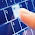## JAVA95

Let's learn java programming language with easy steps. This Java tutorial provides you complete knowledge about java technology.

# Java 8 Lambda Expressions Tutorial with Examples

### Java 8 Lambda Expressions Tutorial

In this article, We are going to cover java 8 lambda expressions tutorial with examples one-by-one in a simple way. Lambda expression is a new feature which introduced in java 8 programming language.

### What is Lambda Expression?

There are many new features introduced in java 8 e.g default and static method in an interface, Array parallel sorting, StringJoiner class, etc. and Lambda expression is one of them which is introduced in java 8 version.

Java 8 lambda expression is used to provide the implementation of the functional interface, An interface which has only one abstract method known as a functional interface.

Lambda expression is anonymous function i.e function with no name. This function does not belong to any class.

Lambda expression concept was first introduced in LISP programming language and the in other programming languages like C,C++,Ruby,Scala,.Net, C#, Java, etc.

Lambda expression is also useful for iterate and extracts the data from the collection.

There are many advantages of lambda expressions and these are given below.
• To enable functional programming in java.
• Lambda expression reduces the length of the code.
• By using lambda expression we write more readable and maintainable code in java.
• To enable parallel processing.

### Syntax of Lambda Expression

(parameter_list) -> {body}

It contains three parts:

Parameter List: Parameter list may be empty or non-empty.

Arrow operator: This is lambda operator which connect the parameter list and body expression.

Body: It contains expression and statements for lambda expressions.

### Java Lambda Expression Example

In this example, we will provide the implementation of function interface by using the lambda expression.

For example:

@FunctionalInterface
interface LambdaExample
{
public void eat();
}
class Test
{
public static void main(String args[])
{
LambdaExample l = () -> {

System.out.println("eat rice");

};
l.eat();
}
}

Output: eat rice

### Java Lambda Expression Example with No Parameter

This is the first java 8 anonymous function example i.e lambda expression example with no parameter.

For example:

@FunctionalInterface
interface MyFunctional
{
public String run();//without parameter
}
class First
{
public static void main(String args[])
{
MyFunctional mf = () -> {

return "fast";
};

System.out.println(mf.run());
}
}

Output: fast

@FunctionInterface is used to declare an interface as the functional interface in java. It is optional.

### Java Lambda Expression Example with One Parameter

This single parameter example of java 8 lambda expression.

For example:

interface My
{
public String watch(String s);
}
class Second
{
public static void main(String args[])
{
My m = (watching) -> {

return "enjoying "+watching;
};
System.out.println(m.watch("cricket"));
}
}

Output: enjoying cricket

### Java Lambda Expression Example with multiple parameters

In this lambda expression example, we will pass two parameters.

For example:

interface My
{
public String hello(String a, String b, String c);
}
class Third
{
public static void main(String args[])
{
My m = (a, b, c) -> a+b+c;
System.out.println(m.hello("I", "am", "good"));
}
}

Output: I am good

### Java Lambda Expression Example with Collection

This is the example of lambda expression with collection and foreach loop.

For example:

import java.util.*;
class LambdaWithCollection
{
public static void main(String args[])
{
List<String> list = new ArrayList<String>();

list.forEach((n) -> System.out.println(n));
}
}

Output: red
black
pink
white
orange

Java Reflection Tutorial with Examples.

Here we have discussed java 8 lambda expression with simple and easy examples.

Share:

1.This is the best java blog for beginners. Thanks for all your useful articles

2.Lambda Expressions is very useful for me.Thanks.
Java Training with Placements

3.I was more than happy to uncover this great site. I need to to thank you for your time due to this fantastic read!! I definitely enjoyed every bit of it and I have you bookmarked to see new information on your blog.
Java Training in Bangalore